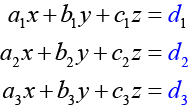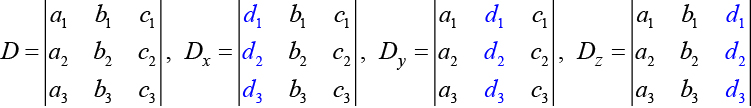11.8 Solving systems with cramer's rule  (Page 3/11)

 Page 3 / 11The algebra is as follows:

$|A|={a}_{1}{b}_{2}{c}_{3}+{b}_{1}{c}_{2}{a}_{3}+{c}_{1}{a}_{2}{b}_{3}-{a}_{3}{b}_{2}{c}_{1}-{b}_{3}{c}_{2}{a}_{1}-{c}_{3}{a}_{2}{b}_{1}$

Finding the determinant of a 3 × 3 matrix

Find the determinant of the 3 × 3 matrix given

$A=\left[\begin{array}{ccc}0& 2& 1\\ 3& -1& 1\\ 4& 0& 1\end{array}\right]$

Augment the matrix with the first two columns and then follow the formula. Thus,

$\begin{array}{l}|A|=|\begin{array}{ccc}0& 2& 1\\ 3& -1& 1\\ 4& 0& 1\end{array}\text{\hspace{0.17em}}\text{\hspace{0.17em}}|\begin{array}{c}0\\ 3\\ \text{\hspace{0.17em}}\text{\hspace{0.17em}}4\end{array}\text{\hspace{0.17em}}\text{\hspace{0.17em}}\text{\hspace{0.17em}}\text{\hspace{0.17em}}\begin{array}{c}2\\ -1\\ 0\end{array}|\hfill \\ \text{\hspace{0.17em}}\text{\hspace{0.17em}}\text{\hspace{0.17em}}\text{\hspace{0.17em}}\text{\hspace{0.17em}}\text{\hspace{0.17em}}\text{\hspace{0.17em}}=0\left(-1\right)\left(1\right)+2\left(1\right)\left(4\right)+1\left(3\right)\left(0\right)-4\left(-1\right)\left(1\right)-0\left(1\right)\left(0\right)-1\left(3\right)\left(2\right)\hfill \\ \text{\hspace{0.17em}}\text{\hspace{0.17em}}\text{\hspace{0.17em}}\text{\hspace{0.17em}}\text{\hspace{0.17em}}\text{\hspace{0.17em}}\text{\hspace{0.17em}}=0+8+0+4-0-6\hfill \\ \text{\hspace{0.17em}}\text{\hspace{0.17em}}\text{\hspace{0.17em}}\text{\hspace{0.17em}}\text{\hspace{0.17em}}\text{\hspace{0.17em}}\text{\hspace{0.17em}}=6\hfill \end{array}$

Find the determinant of the 3 × 3 matrix.

$\mathrm{det}\left(A\right)=|\begin{array}{ccc}1& -3& 7\\ 1& 1& 1\\ 1& -2& 3\end{array}|$

$-10$

Can we use the same method to find the determinant of a larger matrix?

No, this method only works for and matrices. For larger matrices it is best to use a graphing utility or computer software.

Using cramer’s rule to solve a system of three equations in three variables

Now that we can find the determinant    of a 3 × 3 matrix, we can apply Cramer’s Rule    to solve a system of three equations in three variables . Cramer’s Rule is straightforward, following a pattern consistent with Cramer’s Rule for 2 × 2 matrices. As the order of the matrix increases to 3 × 3, however, there are many more calculations required.

When we calculate the determinant to be zero, Cramer’s Rule gives no indication as to whether the system has no solution or an infinite number of solutions. To find out, we have to perform elimination on the system.

Consider a 3 × 3 system of equations.$x=\frac{{D}_{x}}{D},y=\frac{{D}_{y}}{D},z=\frac{{D}_{z}}{D},D\ne 0$

whereIf we are writing the determinant $\text{\hspace{0.17em}}{D}_{x},$ we replace the $\text{\hspace{0.17em}}x\text{\hspace{0.17em}}$ column with the constant column. If we are writing the determinant ${D}_{y},$ we replace the $\text{\hspace{0.17em}}y\text{\hspace{0.17em}}$ column with the constant column. If we are writing the determinant $\text{\hspace{0.17em}}{D}_{z},$ we replace the $\text{\hspace{0.17em}}z\text{\hspace{0.17em}}$ column with the constant column. Always check the answer.

Solving a 3 × 3 system using cramer’s rule

Find the solution to the given 3 × 3 system using Cramer’s Rule.

$\begin{array}{c}x+y-z=6\\ 3x-2y+z=-5\\ x+3y-2z=14\end{array}$

Use Cramer’s Rule.

$D=|\begin{array}{ccc}1& \text{\hspace{0.17em}}\text{\hspace{0.17em}}1& -1\\ 3& -2& \text{\hspace{0.17em}}\text{\hspace{0.17em}}\text{\hspace{0.17em}}1\\ 1& \text{\hspace{0.17em}}\text{\hspace{0.17em}}3& -2\end{array}|,{D}_{x}=|\begin{array}{ccc}6& 1& -1\\ -5& -2& \text{\hspace{0.17em}}\text{\hspace{0.17em}}\text{\hspace{0.17em}}1\\ 14& \text{\hspace{0.17em}}\text{\hspace{0.17em}}3& -2\end{array}|,{D}_{y}=|\begin{array}{ccc}1& \text{\hspace{0.17em}}6& -1\\ 3& -5& \text{\hspace{0.17em}}\text{\hspace{0.17em}}1\\ 1& 14& -2\end{array}|,{D}_{z}=|\begin{array}{ccc}1& \text{\hspace{0.17em}}1& 6\\ 3& -2& -5\\ 1& \text{\hspace{0.17em}}\text{\hspace{0.17em}}3& 14\end{array}|$

Then,

$\begin{array}{l}x=\frac{{D}_{x}}{D}=\frac{-3}{-3}=1\hfill \\ y=\frac{{D}_{y}}{D}=\frac{-9}{-3}=3\hfill \\ z=\frac{{D}_{z}}{D}=\frac{6}{-3}=-2\hfill \end{array}$

The solution is $\left(1,3,-2\right).$

Use Cramer’s Rule to solve the 3 × 3 matrix.

$\begin{array}{r}\hfill x-3y+7z=13\\ \hfill x+y+z=1\text{\hspace{0.17em}}\text{\hspace{0.17em}}\text{\hspace{0.17em}}\\ \hfill x-2y+3z=4\text{\hspace{0.17em}}\text{\hspace{0.17em}}\text{\hspace{0.17em}}\end{array}$

$\left(-2,\frac{3}{5},\frac{12}{5}\right)$

Using cramer’s rule to solve an inconsistent system

Solve the system of equations using Cramer’s Rule.

$\begin{array}{l}3x-2y=4\text{ }\left(1\right)\\ 6x-4y=0\text{ }\left(2\right)\end{array}$

We begin by finding the determinants

$D=|\begin{array}{cc}3& -2\\ 6& -4\end{array}|=3\left(-4\right)-6\left(-2\right)=0$

We know that a determinant of zero means that either the system has no solution or it has an infinite number of solutions. To see which one, we use the process of elimination. Our goal is to eliminate one of the variables.

1. Multiply equation (1) by $\text{\hspace{0.17em}}-2.$
2. Add the result to equation $\text{\hspace{0.17em}}\left(2\right).$
$\begin{array}{l}\underset{_______________}{\begin{array}{l}\begin{array}{l}\hfill \\ -6x+4y\text{\hspace{0.17em}}\text{\hspace{0.17em}}\text{\hspace{0.17em}}\text{\hspace{0.17em}}=-8\hfill \end{array}\hfill \\ \text{\hspace{0.17em}}\text{\hspace{0.17em}}\text{\hspace{0.17em}}6x-4y\text{\hspace{0.17em}}\text{\hspace{0.17em}}\text{\hspace{0.17em}}\text{\hspace{0.17em}}\text{\hspace{0.17em}}\text{\hspace{0.17em}}=\text{\hspace{0.17em}}\text{\hspace{0.17em}}\text{\hspace{0.17em}}\text{\hspace{0.17em}}0\hfill \end{array}}\hfill \\ \text{\hspace{0.17em}}\text{\hspace{0.17em}}\text{\hspace{0.17em}}\text{\hspace{0.17em}}\text{\hspace{0.17em}}\text{\hspace{0.17em}}\text{\hspace{0.17em}}\text{\hspace{0.17em}}\text{\hspace{0.17em}}\text{\hspace{0.17em}}\text{\hspace{0.17em}}\text{\hspace{0.17em}}\text{\hspace{0.17em}}\text{\hspace{0.17em}}\text{\hspace{0.17em}}\text{\hspace{0.17em}}\text{\hspace{0.17em}}\text{\hspace{0.17em}}\text{\hspace{0.17em}}0\text{\hspace{0.17em}}\text{\hspace{0.17em}}\text{\hspace{0.17em}}\text{\hspace{0.17em}}\text{\hspace{0.17em}}\text{\hspace{0.17em}}=\text{\hspace{0.17em}}-8\hfill \end{array}$

We obtain the equation $\text{\hspace{0.17em}}0=-8,\text{\hspace{0.17em}}$ which is false. Therefore, the system has no solution. Graphing the system reveals two parallel lines. See [link] .

Use cramer’s rule to solve a dependent system

Solve the system with an infinite number of solutions.

$\begin{array}{rr}\hfill x-2y+3z=0& \hfill \left(1\right)\\ \hfill 3x+y-2z=0& \hfill \left(2\right)\\ \hfill 2x-4y+6z=0& \hfill \left(3\right)\end{array}$

Let’s find the determinant first. Set up a matrix augmented by the first two columns.

Then,

$1\left(1\right)\left(6\right)+\left(-2\right)\left(-2\right)\left(2\right)+3\left(3\right)\left(-4\right)-2\left(1\right)\left(3\right)-\left(-4\right)\left(-2\right)\left(1\right)-6\left(3\right)\left(-2\right)=0$

As the determinant equals zero, there is either no solution or an infinite number of solutions. We have to perform elimination to find out.

1. Multiply equation (1) by $\text{\hspace{0.17em}}-2\text{\hspace{0.17em}}$ and add the result to equation (3):
$\frac{\begin{array}{r}\hfill -2x+4y-6x=0\\ \hfill 2x-4y+6z=0\end{array}}{\text{\hspace{0.17em}}\text{\hspace{0.17em}}\text{\hspace{0.17em}}\text{\hspace{0.17em}}\text{\hspace{0.17em}}\text{\hspace{0.17em}}\text{\hspace{0.17em}}\text{\hspace{0.17em}}\text{\hspace{0.17em}}\text{\hspace{0.17em}}\text{\hspace{0.17em}}\text{\hspace{0.17em}}\text{\hspace{0.17em}}\text{\hspace{0.17em}}\text{\hspace{0.17em}}\text{\hspace{0.17em}}\text{\hspace{0.17em}}\text{\hspace{0.17em}}\text{\hspace{0.17em}}\text{\hspace{0.17em}}\text{\hspace{0.17em}}\text{\hspace{0.17em}}\text{\hspace{0.17em}}\text{\hspace{0.17em}}\text{\hspace{0.17em}}\text{\hspace{0.17em}}\text{\hspace{0.17em}}\text{\hspace{0.17em}}\text{\hspace{0.17em}}\text{\hspace{0.17em}}\text{\hspace{0.17em}}0=0}$
2. Obtaining an answer of $\text{\hspace{0.17em}}0=0,\text{\hspace{0.17em}}$ a statement that is always true, means that the system has an infinite number of solutions. Graphing the system, we can see that two of the planes are the same and they both intersect the third plane on a line. See [link] .

The sequence is {1,-1,1-1.....} has
how can we solve this problem
Sin(A+B) = sinBcosA+cosBsinA
Prove it
Eseka
Eseka
hi
Joel
June needs 45 gallons of punch. 2 different coolers. Bigger cooler is 5 times as large as smaller cooler. How many gallons in each cooler?
7.5 and 37.5
Nando
find the sum of 28th term of the AP 3+10+17+---------
I think you should say "28 terms" instead of "28th term"
Vedant
the 28th term is 175
Nando
192
Kenneth
if sequence sn is a such that sn>0 for all n and lim sn=0than prove that lim (s1 s2............ sn) ke hole power n =n
write down the polynomial function with root 1/3,2,-3 with solution
if A and B are subspaces of V prove that (A+B)/B=A/(A-B)
write down the value of each of the following in surd form a)cos(-65°) b)sin(-180°)c)tan(225°)d)tan(135°)
Prove that (sinA/1-cosA - 1-cosA/sinA) (cosA/1-sinA - 1-sinA/cosA) = 4
what is the answer to dividing negative index
In a triangle ABC prove that. (b+c)cosA+(c+a)cosB+(a+b)cisC=a+b+c.
give me the waec 2019 questions
the polar co-ordinate of the point (-1, -1)ByByBy Lakeima RobertsByBy Dravida Mahadeo-J...